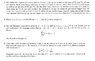# Several Induction questions

• moocav
In summary, the conversation includes a student seeking help with two induction questions. The first question involves finding a pattern in numbers and the second question involves showing that a function is positive and its numerator is less than a given number. The student also shares their attempts at solving the problems and asks for guidance.

#### moocav

2 Induction Questions

## Homework Statement

I have quite a few questions and so i just made it an image. Also attached.
http://img411.imageshack.us/img411/1002/inductionforlife.jpg" [Broken]
Only need help with questions 2 and 5 now

Oh and so far my lecturer has taught well-ordering, strong induction and simple induction. But I could only follow simple induction... the other two I'm quite clueless about >< Though tell me which method is best for each question.

## Homework Equations

Refer to image

3. The Attempt at a Solution s
Question 2
I have no clue to how to start it..
All i have done is
24 = 7 + 7 + 5 + 5
25 = 5 + 5 + 5 + 5 + 5
26 = 7 + 7 + 7 + 5
27 = 7 + 5 + 5 + 5 + 5
28 = 7 + 7 + 7 + 7
29 = 7 + 7 + 5 + 5 + 5
no idea what to do now

[STRIKE]Question 3
I'm not sure if my method is correct but I've proved that when x = 0 and n = 1, x = 1 n = 2 are true. But I get stuck whilst proving n = k + 1

Let Sn be (1 + x)n >= 1 + nx
For n = 1 and x = 0, S1 =
LHS = (1+0)1 = 1
RHS = 1 + (1)(0) = 1
Therfore LHS >= RHS Hence n = 1 is true.

Assume n = k is true
Sk --> (1 + x)k >= 1 + kx

For n = k + 1, Sk+1 =

I know that I need to get to
(1 + x)k+1 >= 1 + (k+1)x

(1 + x)k >= 1 + kx
(1 + x)k(1 + x)1 >= (1 + kx)(1 + x)1 (multiplied both sides by (x + 1)
(1 + x)k+1 >= 1 + x + kx + kx2

I can see that on the RHS there is 1 + x + kx I'm not sure what to do with it... hints/help?

Question 4 (Just needs checking)

Let Sn be ƒ1 + ƒ2 + ... + ƒn = ƒn+2 -1
For n = 1, S1
LHS = ƒ1 = 1
RHS = ƒ1+2 - 1 = 2 - 1 = 1
LHS = RHS
Therefore n = 1 is true

Assume true for n = k
Sk --> ƒ1 + ƒ2 + ... + ƒk = ƒk+2 -1

For n = k + 1, Sk+1 =
RHS = ƒk+3 - 1
LHS = ƒ1 + ƒ2 + ... + ƒk + ƒk+1
= ƒk+2 -1 + ƒk+1
= ƒk+2 + ƒk+1 - 1
= ƒk+3 - 1 (should I write any reason here? if yes..what should i write?)
= RHS
Hence n = k + 1 is true
By mathematical induction Sn is true for all positive integers n.
[/STRIKE]
Question 5
Show that n/t - 1/(q+1) is positive and numerator is less than n
where t = nq + r with 0 < r < n

(get common denominator then expand and simplify)
n/t - 1/(q+1)
= n(q + 1)/[t(q+1)] - t/[t(q+1)]
= [n(q+1) - t] / [t(q+1)]
= [nq - t + n] / [t(q+1)]

t = nq + r
nq - t = -r

hence n/t - 1/(q+1)
= [n-r] / [t(q+1)]

from 0 < r < n
n > r therefore n - r > 0 (proved that numerator is positive)
and since r > 0 then n - r < n (proved that numerator is < n)

I'm not sure where to go from here

Please someone help me however you can..

#### Attachments

•induction for life.JPG
53.8 KB · Views: 394
Last edited by a moderator:
Bump (updated)

It's better to post one question at a time rather than post a whole slew of them.

Mark44 said:
It's better to post one question at a time rather than post a whole slew of them.

Its only two questions now ><
and they don't have to answer them all...just whichever ones they can ><

Last edited: